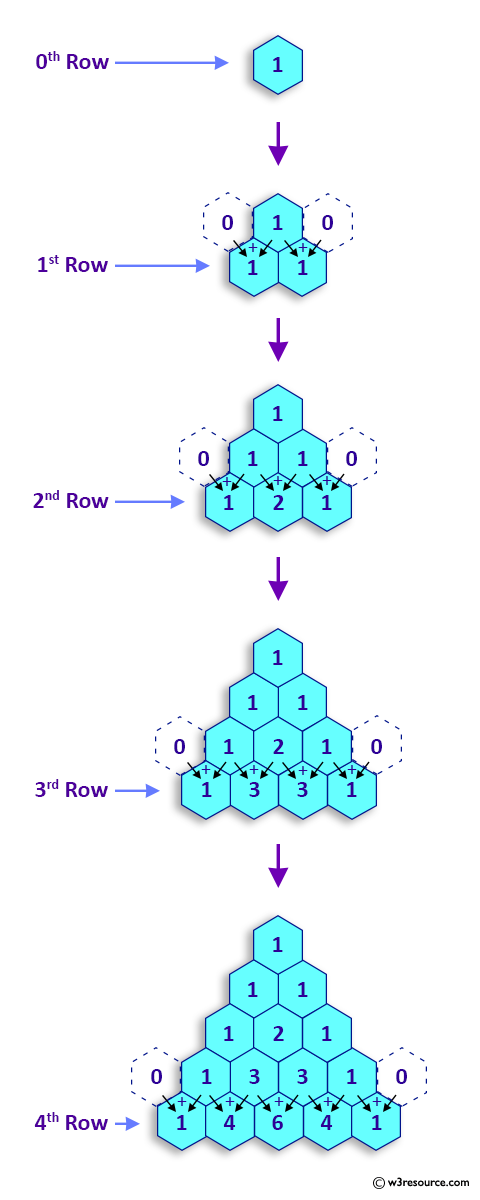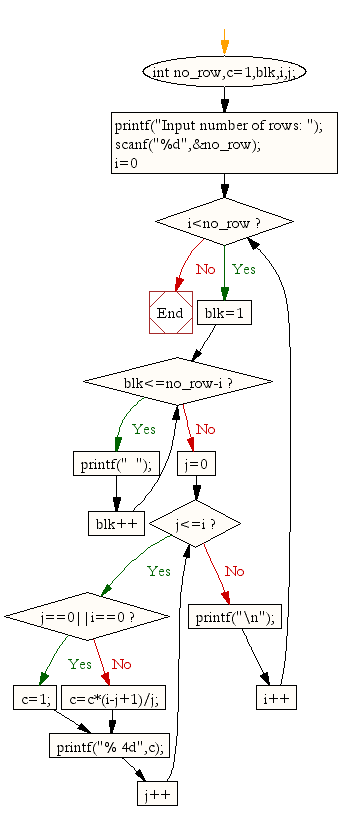﻿ C Program: Display Pascal's triangle - w3resource

# C Exercises: Display Pascal's triangle

## C For Loop: Exercise-33 with Solution

Write a C program to display Pascal's triangle.

Construction of Pascal's Triangle:

As shown in Pascal's triangle, each element is equal to the sum of the two numbers immediately above it.Sample Solution:

C Code:

``````#include <stdio.h> // Include the standard input/output header file.

int main() {
int no_row, c = 1, blk, i, j; // Declare variables for row count, pattern counter, and loop control.

// Prompt the user to input the number of rows.
printf("Input number of rows: ");
scanf("%d", &no_row); // Read the input from the user.

// Outer loop for iterating over rows.
for (i = 0; i < no_row; i++) {
// Inner loop for printing spaces.
for (blk = 1; blk <= no_row - i; blk++)
printf("  ");

// Inner loop for generating and printing pattern.
for (j = 0; j <= i; j++) {
if (j == 0 || i == 0) // If it's the first column or first row, set c to 1.
c = 1;
else
c = c * (i - j + 1) / j; // Calculate the next pattern value.
printf("% 4d", c); // Print the pattern value.
}

printf("\n"); // Move to the next row.
}

return 0; // Return 0 to indicate successful execution.
}

```
```

Sample Output:

```Input number of rows: 5
1
1   1
1   2   1
1   3   3   1
1   4   6   4   1
```

Flowchart:C Programming Code Editor:

What is the difficulty level of this exercise?

Test your Programming skills with w3resource's quiz.

﻿